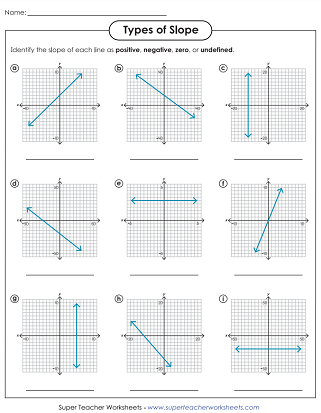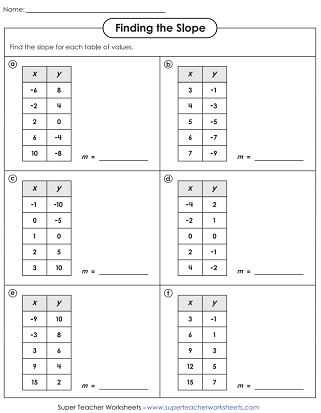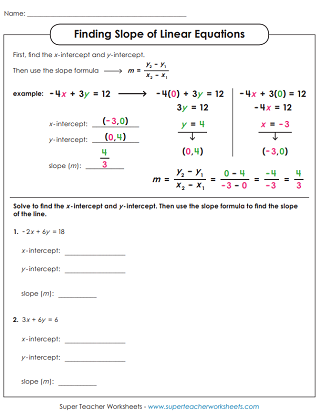# Finding Slope

On these printable worksheets, students are given ordered pairs or a graph and are instructed to find the slope. These worksheets align with Common Core CCSS.8.EE.5.## Slope(Ordered Pairs)

Students are given ordered pairs. They use them to calculate the slope, using the rise over run formula. This is a two-page worksheet. An example is given at the top of the first page.
Students are presented with ordered pairs. They calculate the slope. This worksheet has space for students to show their work.

## Slope(Graphs)

Students are shown graphs of slopes. Students determine the ordered pairs of the points shown. Then they use the ordered pairs to calculate the slope of the line.

## Slope(X / Y Tables)

On this worksheet, students are shown x/y value tables. They use the numbers on the tables to calculate the slopes of the lines.

## Linear Equation(Y-Intercept Slope)

Solve to find the x-intercept and y-intercept. Then use the slope formula to find the slope of the line.

## 4 Types of Slope

Tell whether each slope is positive, negative, undefined, or zero.
For each task card, students will determine the type of slope shown. Choose from positive, negative, zero, or undefined.

## Mixed Slope Problems

Find the slope for each problem. 2 problems give coordinate pairs. Two problems show a graph. Two problems have an x/y value table.

## Reference Chart

Students can glue this anchor chart into their notebooks. It shows the four types of slope, and shows how to calculate the slope of a line.
More Middle School Math Topics

We have a printables on a variety of middle school math topics.

## Sample Worksheet ImagesMy Account
Site Information